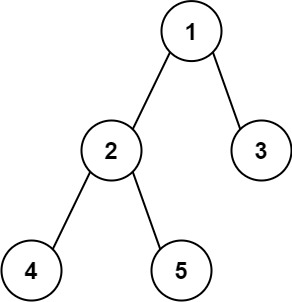### LeetCode• ㊗️
• 大家
• offer
• 多多！

## Problem

Given the root of a binary tree, return the length of the diameter of the tree.

The diameter of a binary tree is the length of the longest path between any two nodes in a tree. This path may or may not pass through the root.

The length of a path between two nodes is represented by the number of edges between them.

Example 1:Input: root = [1,2,3,4,5]
Output: 3
Explanation: 3is the length of the path [4,2,1,3] or [5,2,1,3].


Example 2:

Input: root = [1,2]
Output: 1


Constraints:

• The number of nodes in the tree is in the range [1, 10^4].
• -100 <= Node.val <= 100

## Code

class Solution {
int max = 0;

public int diameterOfBinaryTree(TreeNode root) {
helper(root);
return max;
}

private int helper(TreeNode root) {
if (root == null) return 0;

int left = helper(root.left);
int right = helper(root.right);

max = Math.max(max, left + right);

return Math.max(left, right) + 1;
}
}

class Solution {
public int diameterOfBinaryTree(TreeNode root) {
if (root == null) return 0;

int left = getHeight(root.left);
int right = getHeight(root.right);

int leftMax = diameterOfBinaryTree(root.left);
int rightMax = diameterOfBinaryTree(root.right);

return Math.max((left + right), Math.max(leftMax, rightMax));
}

private int getHeight(TreeNode root) {
if (root == null) return 0;

return 1 + Math.max(getHeight(root.left), getHeight(root.right));
}
}